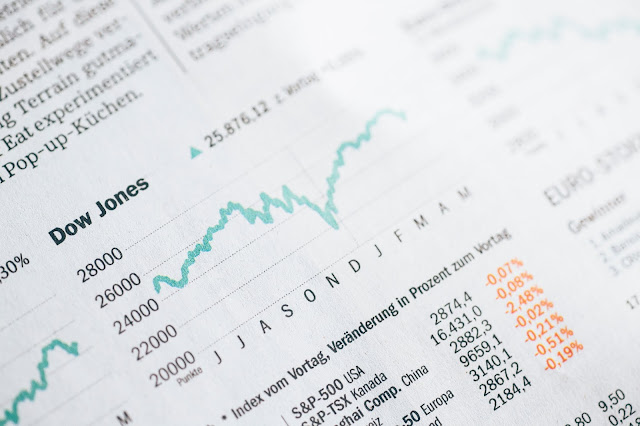## Posts

Showing posts from January, 2020

### Problems with spreadsheetsBy McDonald, T.   |  Updated 24th of March 2020 Small errors in spreadsheets have been the cause of major problems in real life. Examining the kinds of common errors in spreadsheets helps us understand the general problems working with data can present. In order to understand these problems, it is a good idea to look at what a spreadsheet is and how equations can go wrong. Spreadsheet ideas   A spreadsheet consists of one or more sheets: A sheet is a two-dimensional (array) of cells in which each cell is referenced by an index consisting of its column letter and row number. A cell can contain data or a calculation (known as a formula). There is a text box, known as a formula bar, in which the contents of a cell are displayed, and which is used for creating and editing the contents of the cell. What is a spreadsheet?   A sheet can contain one or more: Tables (sheets) A table is a two-dimensional array of data; some data is numeric, some is text. The tabl